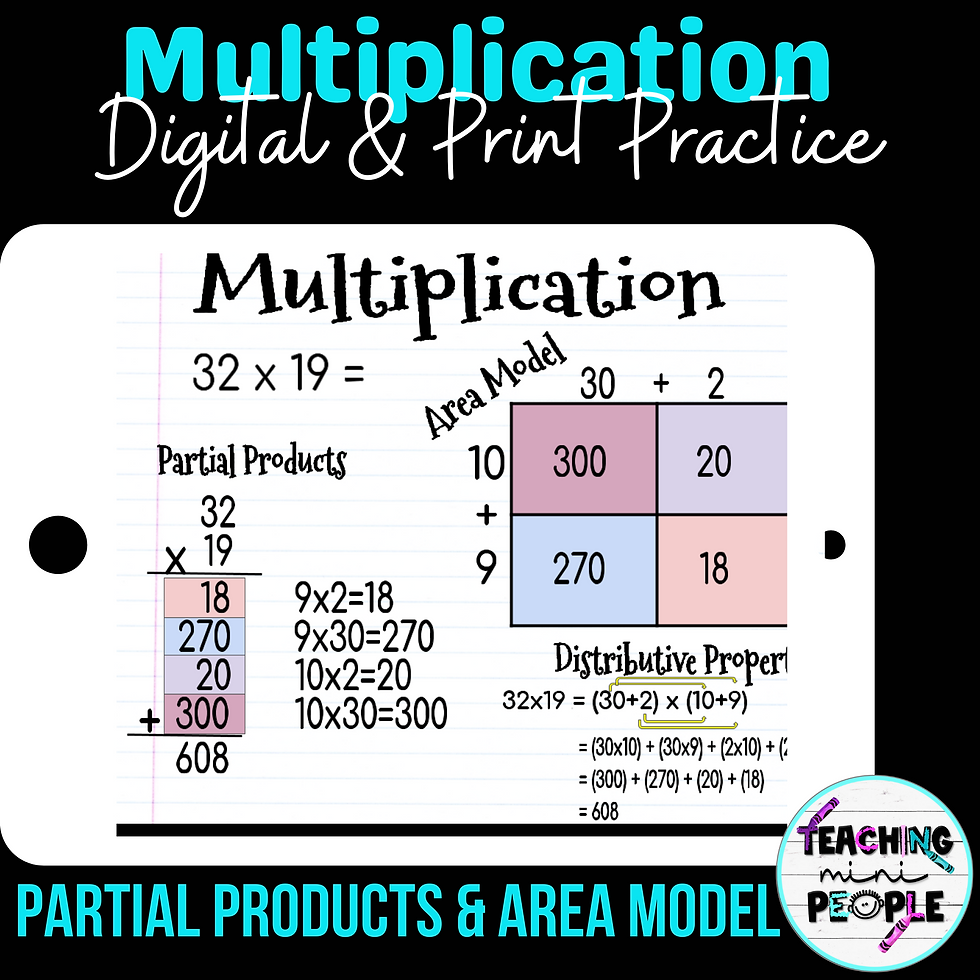top of page
Search
• Kristy Johnson

# How to Teach Partial Products in Multiplication## What is a Partial Product Model?

A partial product model in multiplication is a term that is typically taught in the 4th and 5th grade math curriculum. This strategy is rooted in number sense understanding. Students use place value concepts to multiply the numbers, making it a strategy that actually enhances number sense, rather than relying on a series of memorized steps.

## What is the Purpose of Partial Products?

Teaching students how to multiply using the partial product method gives them a more pictorial and concrete understanding of the process of multiplying 2 digit numbers or higher. Using the partial products method will make sure to give them a conceptual understanding of multi-digit multiplication before moving to the standard method of say just multiplying 43 X 56.

When children use the standard method of multiplying multi-digit numbers, it is a much more abstract concept so they MUST have a solid understanding of partial products. This video does a really great job of explaining this!## What are the Steps of Teaching Partial Products?

### Here is how I teach the Partial Products Method in Multiplication to students:

1. Determine the value of each digit.

4. Finally, add the partial products together!

## Partial Products Multiplication Example & Tip

Here is an example of how I teach my students to use partial products in multiplication:

### EXAMPLE: 257 X 3= ?

Determine the value of each digit then write your multiplication sentences:So, the partial products are 600 + 150 + 21 and the answer to this problem is 771.

## Partial Products Multiplication Tip

I always teach my students this tip: When determining how many multiplication sentences and partial products you will have, you can multiply your first number of digits by your second number of digits.

For example: 467 x 5

Your first number has 3 digits and your second number has 1 digit, therefore, you will have 3 partial products to add up.

If your problem is 23 x 79: your first number has 2 digits and your second number has 2 digits. Multiply 2 x 2 together and you will have 4 partial products to add up. This is a great tip for them to check their work and make sure they are on the right track with how many addition sentences they have!

## Partial Products Multiplication Anchor Chart

Below is an example of an anchor chart that I like to use with my students when teaching about partial products. It really helps them to have a visual representation available in the classroom of each step for when we do our practice and independent work.## The Multiplication Area Model

There is also a follow-up method to teaching partial products in multiplication to students. It's called the Area Model in multiplication. Sometimes with my students, I refer to it as the Box & Cluster Method.

### Here is an example of what the Area Model (or Box & Cluster Method) looks like:## Activities for Teaching Partial Products and the Area Method

When teaching students how to find partial products, we always work through these Partial Products Notes and Practice together in class. This is the perfect introduction activity for teaching partial products in multiplication to your students. There are 4 digit x 1 digit multiplication problems to work through and even 3 digit x 2 digit practice problems. Plus you can print it out or use it digitally with your students through Google Slides.## GRAB THIS PARTIAL PRODUCTS NOTES AND PRACTICE ACTIVITY BY CLICKING HERE

To follow up with MORE PRACTICE ON PARTIAL PRODUCTS, we use this Partial Products for Multiplication Activity. It has 14 practice problems to work through, using both partial products and the area model. It can be printed out to use with students or used digitally.